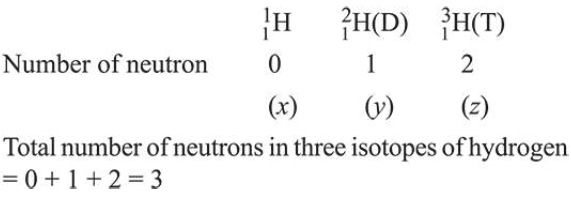# Hydrogen has three isotopes (A),(B) and (C).

Question:

Hydrogen has three isotopes $(A),(B)$ and $(C)$. If the number of neutron(s) in (A), (B) and (C) respectively, are $(x),(y)$ and $(z)$, the sum of $(x),(y)$ and $(z)$ is:

1. 3

2. 2

3. 4

4. 1

Correct Option:

Solution: